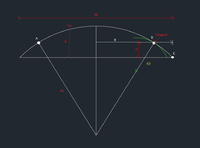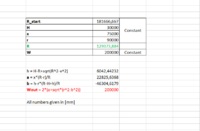# Changing the radius of an arch so its length is the same, but its width is smaller

#### Severin

##### New member
Hello.
This is a pretty tricky question, but I hope you can help me.
Background: In the figure you can see an arch. The task is to decrease the radius at the end of the arch (green arch). The problem is when the radius is decreased the length of the arch (W) will be shorter. I want this length (W) to be constant. Therefore the radius (R1) between point A and B needs to be increased in order "move" the green arch so that it hits point C.
Also the green arch has to be tangential to the point B
So the question is: "Is there a way to figure out how much R1 has to increase in order for the green arch to hit C?"

Data:
H = constant
h = constant
W = constant
B can vary along X#### Jomo

##### Elite Member
What have you tried? Where are you stuck? We canhelp but we need to know where you need help. Thanks.

#### Severin

##### New member
I’m actually really not sure where to begin, so I have not started on it.
Thank you for helping

#### Dr.Peterson

##### Elite Member
I have been playing with the problem a little, and realized that it is underspecified: there will be infinitely many pairs (R1, R2) that will work; in fact, for any location of B (within some domain) there will be such a pair; or, for any R2 (again, within reason), we could find an R1.

Is there an additional constraint? For instance, is R2 given?

#### Severin

##### New member
Thank you so much for looking into it! I'm sorry I didn't specify it, but yes R2 is given and should be constant.

#### Dr.Peterson

##### Elite Member
Okay, I'd started to think that was most likely.

I've also done enough work on it to strongly suspect that, like some other problems I've tried recently, this might end up with a fourth degree equation, and would not be solvable in terms of a formula. If we need to use some technology to come up with a numerical solution, what sort of thing would be most appropriate? That might be design software, or a spreadsheet, or a graphing calculator or computer algebra program. I've experimented with it, for example, on GeoGebra.

#### Severin

##### New member
Yes I thought it might be tricky to solve by hand :O That would be perfect if you could help me find a numerical solution! I have some experience with GeoGebra and a little bit with Mathlab. Spreadsheets like Excel I have good experience with. So it's up to you what program you choose. I'm just glad you want to help#### Dr.Peterson

##### Elite Member
I'll see what I can do in making a set of equations suitable for solving with Excel. WolframAlpha is another possibility I'll try.

Just as a check of what you need, will it be enough just to be able to enter one set of specific numbers, do a couple commands (such as Solve), and get the value of R1? (That is, solving one specific case at a time, as opposed to being able to make a table of many values at once, or a graph.)

By the way, we usually consider this site to be a place to help people learn to solve problems themselves, so we don't give actual solutions; but this problem seems to complicated to take that approach. My main goal is to find an equation that you can then give to software to solve, the latter being relatively easy.

#### Dr.Peterson

##### Elite Member
I've got a spreadsheet that seems to work, using Goal Seek.

I give it H, h, and r, then use a guessed R to calculate x, the distance from your centerline to B, and (a,b), the coordinates of the center of the smaller arc relative to origin at the midpoint of the chord, and finally W, using these formulas (based on a couple right triangles and a pair of similar triangles):

x = sqrt((2R - H + h)(H - h))​
a = x(R - r)/R​
b = h - r(R - H + h)/R​
W = 2(a + sqrt(r^2 - b^2))​

Then I use Goal Seek to make W equal the desired number by changing R. It gave the correct values for everything for at least one construction on GeoGebra.

•MarkFL

#### Severin

##### New member
I'll see what I can do in making a set of equations suitable for solving with Excel. WolframAlpha is another possibility I'll try.

Just as a check of what you need, will it be enough just to be able to enter one set of specific numbers, do a couple commands (such as Solve), and get the value of R1? (That is, solving one specific case at a time, as opposed to being able to make a table of many values at once, or a graph.)

By the way, we usually consider this site to be a place to help people learn to solve problems themselves, so we don't give actual solutions; but this problem seems to complicated to take that approach. My main goal is to find an equation that you can then give to software to solve, the latter being relatively easy.
The solution you are suggesting sounds very good. It will not be necessary to solve more than one case at the time. So a solver that can give a specific R1 would be perfect.
Thank you so much for your help. It’s much appreciated.

#### Severin

##### New member
Thank you so much for your interest in this.
Still some questions:
• In the calculations do you use r=R2 and R=R1 from the attachment I put out?
• I'm unfamiliar with using goal seek. Is it possible for you to show me how you set up this using goal seek? That would be of so much help.

#### Dr.Peterson

##### Elite Member
•Severin

#### Severin

##### New member
Wow, it works like a charm. I can't thank you enough for this!#### Severin

##### New member
Hey again @Dr.Peterson
I was wondering if it's possible to do a simular calculation but now to keep the distance (x) constant and the hight (h) as a variable?

#### Dr.Peterson

##### Elite Member
I just tried a quick fix, replacing an equation for x with an equation for h, and things seem to become unstable. I'll have to try redoing the whole set of equations with the new goal in mind, when I have time.

#### Severin

##### New member
Hey, I just wondered if you have had look at it? I don't mean to be bother you, but I'm on a bit of a tight time scedule. I'm really grateful that you help me#### Severin

##### New member
Hey. I tried using thos method to solve the problem, but it seems that it doesn't work. Maybe I explained it poorly the last time. I think that in order for it to work the equations must somehow be set up so that we get a Hout, instead of Wout, and then use goal seek to change Hout to H, by changing R. W can here be a constant, like H was in the previous problem.

#### Dr.Peterson

##### Elite Member
Please tell me what you are doing, and what happens, so I can see what exactly is not working. What inputs do you give, and what outputs or error messages do you get? It worked for me, but maybe your numbers are sufficiently different that it behaves differently.

And is there a specific reason you think we should be seeking H rather than W, or is that just a wild guess? (W is a constant in my spreadsheet, just not used as an input to a formula, but as a goal.)

#### Severin

##### New member
Okey, so my goal is to create an arch bridge which is 200m long (W) and 30m hight (H) (all the numbers in the spreadsheet are given in millimeters). R_start is the radius of the arch when the arch is part of a circle with the corresponding dimensions given above.

If we say that r=90000mm and x=75000mm like in the spreadsheet, we get a corresponding value for R to be 129373,883mm after using goal seek. If I then try to model this arch, I find that it doesn't fit, that's because for any given number (r) the value for (R) is never larger than R_start, which it must be.

Maybe I'm wrong about seeking H. I was just thinking it would make sense since this problems is sort of "opposite" from the previous problem.